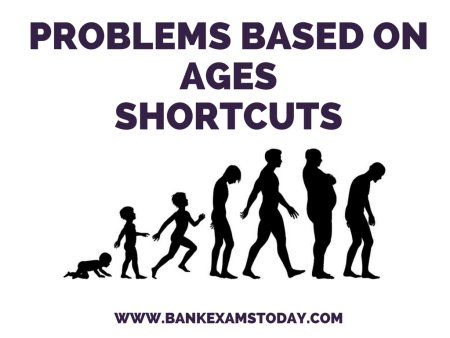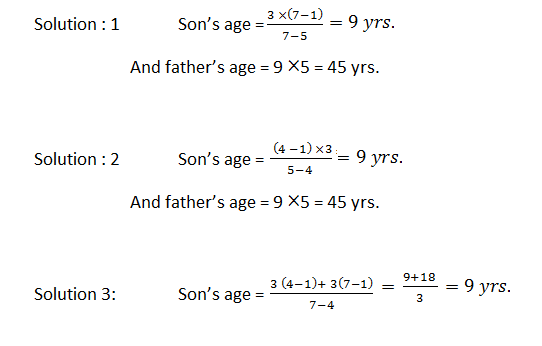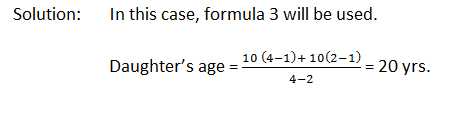New Students Offer - Use Code HELLO

# Problems based on Ages - Tricks

To solve the problems based on ages, you need to have knowledge of linear equations. This method needs some basic concepts as well as some more time than it deserves. Sometimes it is easier to solve the problems by taking the given choices in account. But this hit-and-trial method proves costly sometimes, when we reach our solution much later. We have tried to evaluate some easier as well as quicker methods to solve this type of questions. Although we are not able to cover each type of questions in this section, our attempt is to minimize your difficulties.

I have already shared a trick related to this chapter here.Have a look at the following questions:

### Ques 1.

The age of the father 3 years ago was 7 times the age of his son. At present the father’s age is five times that of his son. What are the present ages of the father and son?

### Ques 2.

At present the age of the father is five times that age of his son.Three years hence, the father’s age would be four times that of his son. Find the present ages of the father and son.

### Ques 3.

Three years earlier the father was 7 times as old as his son. Three years hence the father’s age would be four times that of his son. What are the present ages of the father and son?

### Solving using Conventional Method:

Solution: 1 Let the present age of son = x yrs.
Then, the present age of father = 5x yrs.

3 years ago, 7(x – 3) = 5x – 3
Or, 7x – 21 = 5x – 3
Or, 2x = 18
Therefore, son’s age = 9 yrs. Fathers’ age = 45 years.

Solution: 2. Let the present age of son = x yrs.

Then, the present age of father = 5x yrs.

3 years hence, 4(x + 3) = 5x + 3
Or, 4x + 12 = 5x + 3
X = 9 yrs.
Therefore, son’s age = 9 yrs. Fathers’ age = 45 years.

Solution: 3. Let the present age of son = x yrs.
And the present age of father = y yrs.
3 yrs earlier

7(x – 3) = y – 3 or, 7x – y = 18……….(i)

3 yrs hence

4(x + 3) = y + 3 or, 4x – y = -9………..(ii)

Solving (i) & (ii) we get, x = 9 yrs & y = 45 yrs.

### Quicker Method:### Ques 4.

10 yrs ago, Sita’s mother was 4 times older than her daughter. After 10 yrs, the mother will be twice older than the daughter. What is the present age of Sita?### Ques 5.

One years ago the ratio between Samir’s age and Ashok’s age was 4 : 3. One year hence the ratio of their ages will be 5 : 4. What is the sum of their present ages in yrs.